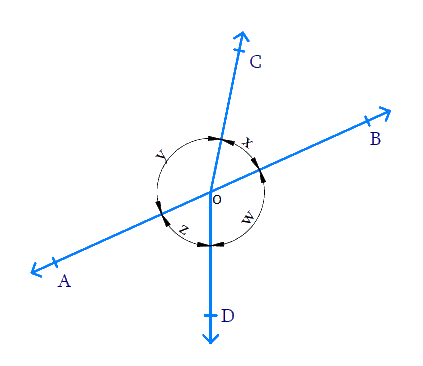In the verge of coronavirus pandemic, we are providing FREE access to our entire Online Curriculum to ensure Learning Doesn't STOP!

# Ex.6.1 Q4 Lines and Angles Solution - NCERT Maths Class 9

Go back to  'Ex.6.1'

## Question

In the given figure, if $$x+y = w+z,$$ then prove that $$AOB$$ is a line.Video Solution
Lines And Angles
Ex 6.1 | Question 4

## Text Solution

What is known?

\begin{align}x+y = w+z\end{align}

What is unknown?

To prove that $$AOB$$ is a line.

Reasoning:

If the sum of two adjacent angles is $$180^ {\circ}$$, then the non–common arms of the angles form a line.

Steps:

From the figure we can see that:

\begin{align}(x + y) +( w + z) = 360^ {\circ} \\ (\text {complete angle})\end{align}

It is given that $$(x + y) = (w + z),$$ Hence

$$(x + y) + (w + z) = 360^ {\circ}$$ can be written as $$(x + y) + (x +y) = 360^ {\circ}$$

\begin{align} 2 x + 2 y &= 360 ^ { \circ } \\ 2 ( x + y ) &= 360 ^ { \circ } \\ x + y &= \frac { 360 } { 2 } \\&= 180 ^ { \circ } \end{align}

Since sum of adjacent angles $$x$$ and $$y$$ with $$OA$$ and $$OB$$ as the non- common arms is $$180^ {\circ}$$ we can say that $$AOB$$ is a line.

Video Solution
Lines And Angles
Ex 6.1 | Question 4

Learn from the best math teachers and top your exams

• Live one on one classroom and doubt clearing
• Practice worksheets in and after class for conceptual clarity
• Personalized curriculum to keep up with school